# The 6 Times Table

The 6 Times Table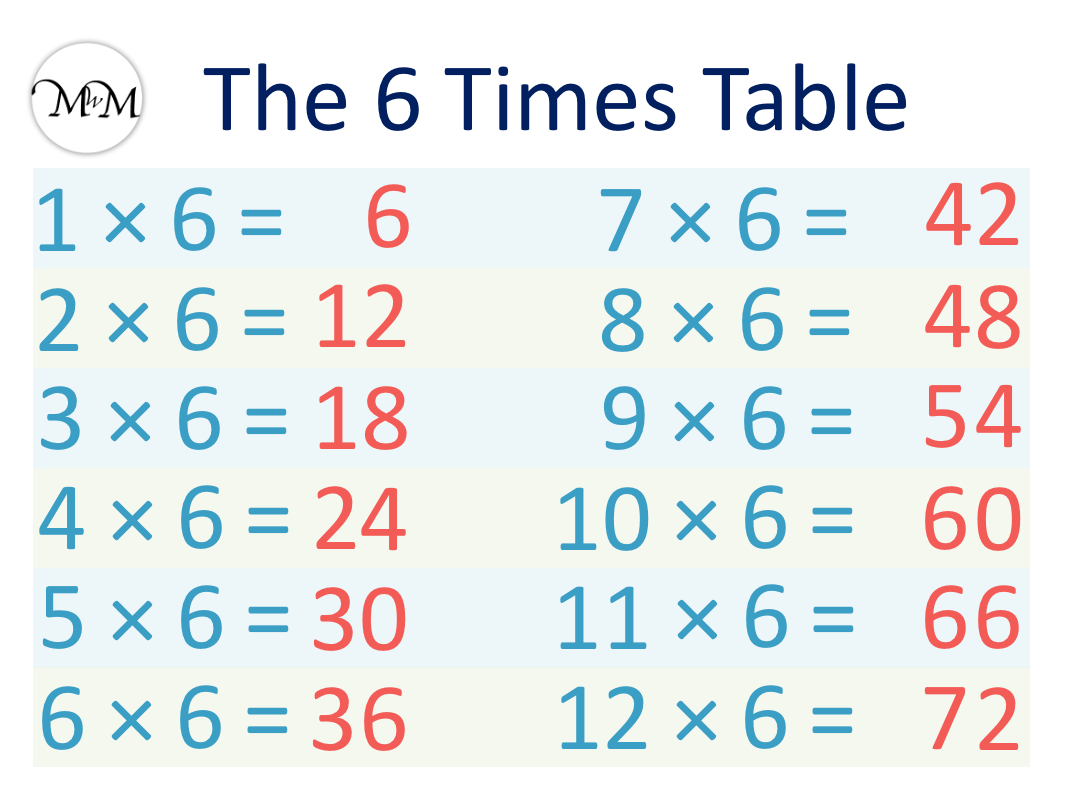The 6 times table is:

• 1 × 6 = 6
• 2 × 6 = 12.
• 3 × 6 = 18
• 4 × 6 = 24
• 5 × 6 = 30
• 6 × 6 = 36
• 7 × 6 = 42
• 8 × 6 = 48
• 9 × 6 = 54
• 10 × 6 = 60
• 11 × 6 = 66
• 12 × 6 = 72• The six times table is made by counting up in sixes.
• The numbers repeat the pattern of ending in 6, 2, 8, 4 and 0.
• We can work out the next number in the six times table by adding a 6 to the previous number.
• When multiplying one of the numbers 2, 4, 6 or 8 by 6, the answer will end in this number and the tens digit will be half of this number.
• When multiplying one of the numbers 3, 5, 7, or 9 by 6, the answer ends in 5 more or less than this number.

The 6 times table repeats the pattern of ending in 6, 2, 8, 4 and 0.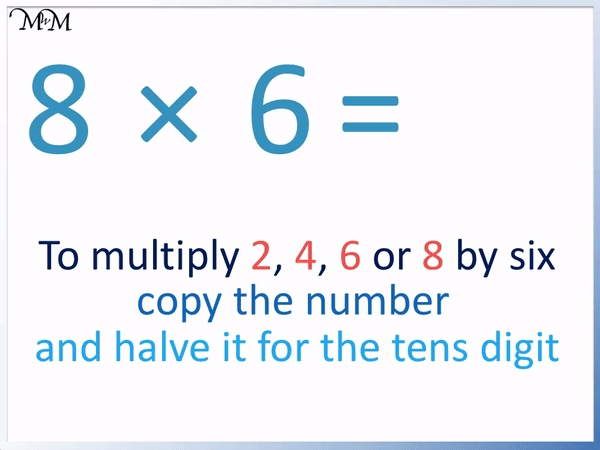• To multiply the numbers 2, 4, 6, or 8 by 6, there is a simple times table trick we can use.
• The answer ends in the same digit. We are multiplying 6 by 8, so our answer ends in an 8.
• The tens digit is half of this.
• Half of 8 is 4 and so, the tens digit is 4.
• 8 × 6 = 48.
• This trick only works when multiplying six by the numbers 2, 4, 6 or 8.#### 6 Times Table Flashcards

Click on the 6 times table flashcards below to memorise the 6 times table.

×
×

=

# The 6 Times Table

## What is the 6 Times Table?

The 6 times table is:

• 1 × 6 = 6
• 2 × 6 = 12
• 3 × 6 = 18
• 4 × 6 = 24
• 5 × 6 = 30
• 6 × 6 = 36
• 7 × 6 = 42
• 8 × 6 = 48
• 9 × 6 = 54
• 10 × 6 = 60
• 11 × 6 = 66
• 12 × 6 = 72

The 6 times table is formed by counting up in sixes.

Below is the 6 times table chart showing the full 6 times table.## How to Learn the 6 Times Table

To learn the 6 times table, remember that the numbers repeat the pattern of ending in 6, 2, 8, 4 and 0. This pattern can help you to add 6 to the previous number to find the next.

Start by memorising 6 multiplied by the numbers 2, 4, 6 and 8. For these entries in the 6 times table, the answer simply ends in the same number and the tens digit is half of this.

Six multiplied by any of the numbers 3, 5, 7 or 9 gives us an answer that ends in 5 more or 5 less than 3, 5, 7 or 9, depending which one you multiplied by.

We will now look further at these tricks for memorising the 6 times table.

The first 6 times table trick is for multiplying the numbers 2, 4, 6 and 8 by six.

There is a pattern in these answers that helps us to memorise them.

When multiplying 6 by any of the numbers 2, 4, 6 or 8, the answer will end in the same number: 2, 4, 6 or 8.

For example in the times table of 2 × 6, the answer will end in 2.

It will start with half of 2. Half of 2 is 1.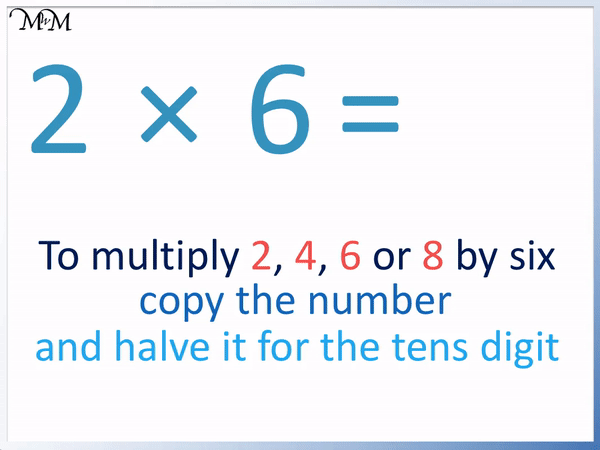We simply write down 2 and then half it to get 1, which we write in front of the 2.

2 × 6 = 12

We can use this times tables trick to multiply six by 4, 6 and 8 in the same way.

Here is the example of 6 × 6.

We simply write down 6 and then halve 6 and write this number in front of the 6.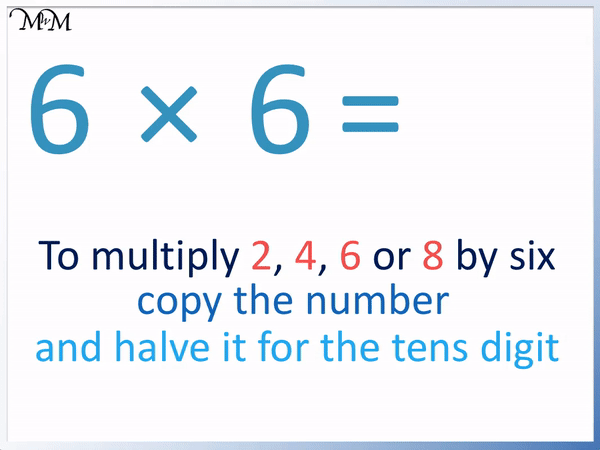Half of 6 is 3 and so our answer begins with a 3.

6 × 6 = 36

Here is another example using the same 6 times table trick.

Here we have 8 × 6.

The answer will end in 8 and begin with half of 8.Half of 8 is 4 and so, the answer will begin with a 4.

In 8 × 6, the answer ends in an 8 and starts with a 4.

8 × 6 = 48

Here is the empty 6 times table chart with the even multiples of 2, 4, 6 and 8 filled in using this times table trick.

When teaching the 6 times table, it is easiest to start by teaching 6 times 2, 4, 6 and 8.This trick works for the even numbers of 2, 4, 6 and 8.

Here is a 6 times table trick for the odd multiples of 6. When multiplying any number of 3, 5, 7 or 9 by 6, the answer will end in a digit that is 5 more or 5 less than the number you multiplied by 6.

Here is the 6 times table chart with the multiples of 3, 5, 7 and 9 shown.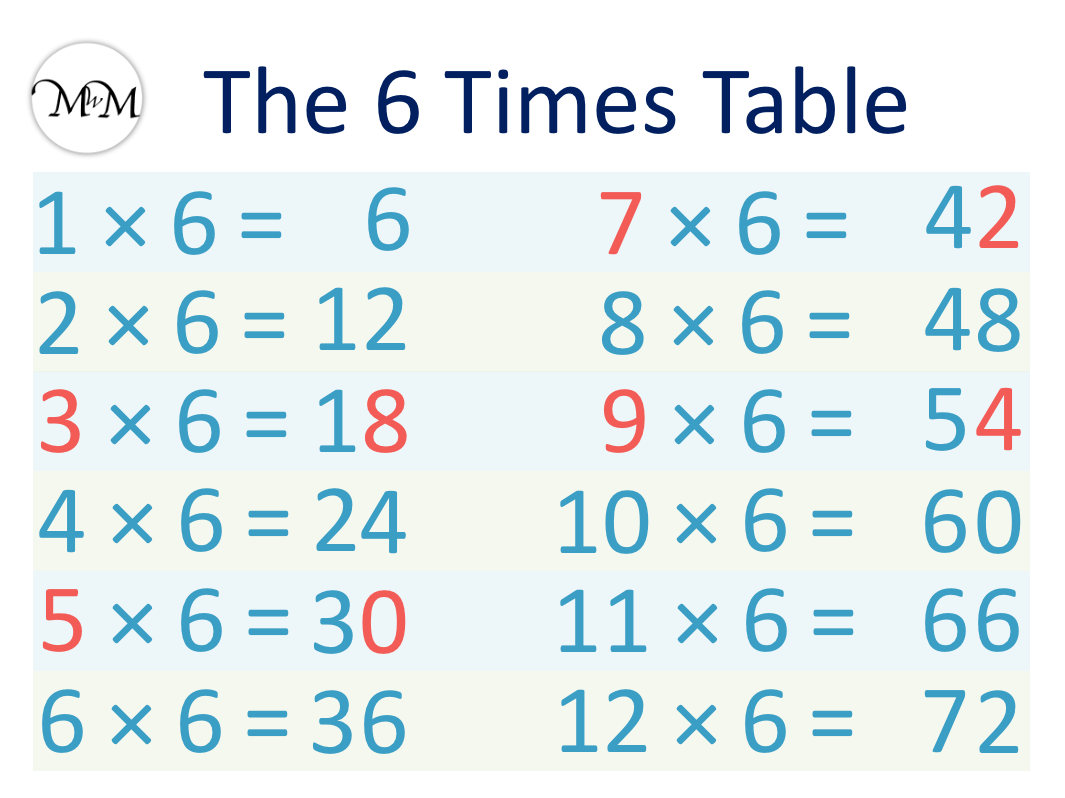If you can subtract 5 from the number 3, 5, 7 or 9, then that is what the answer ends in.

Otherwise, add 5 to the number 3, 5, 7 or 9 and that is what the answer ends in.

For example, here is 3 × 6.

It is easier to add 5 to 3 than to subtract 5 and so, we add 5 to 3 to see what 3 × 6 ends in.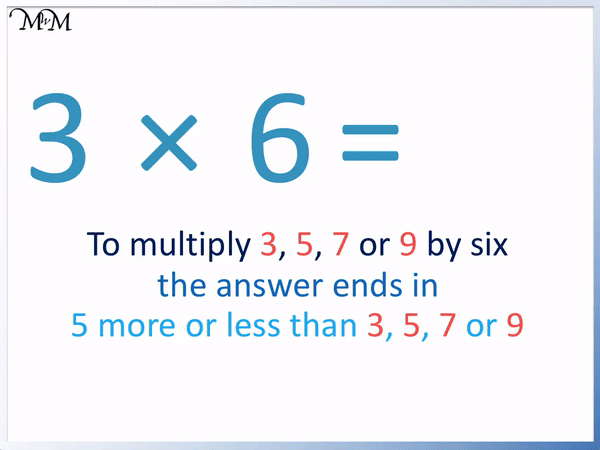3 + 5 = 8 and so, 3 × 6 ends in an 8.

This can help us remember that 3 × 6 = 18.

Here is 5 × 6.

We can subtract 5 from 5 to get 0 to see that our answer will end in a zero. We could also add 5 to 5 to get 10, which also ends in a 0.

The answer to 5 × 6 will end in a zero.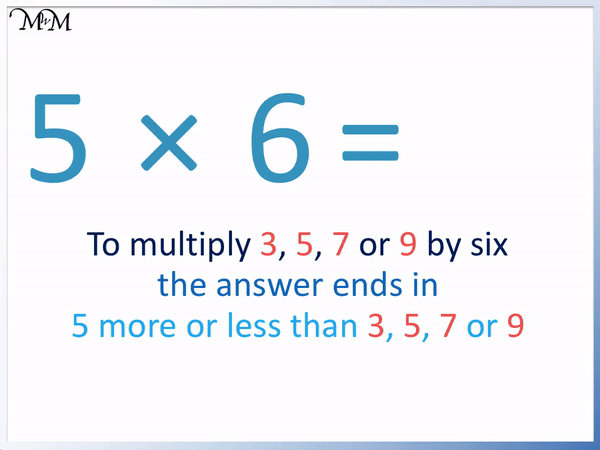We can see that 5 × 6 = 30.

Here is 7 × 6.

We can subtract 5 from 7 to see what 7 × 6 ends in.

7 – 5 = 2 and so, 7 × 6 ends in a 2.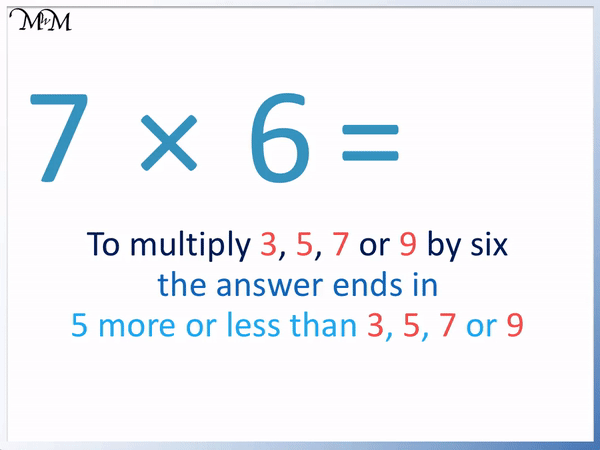We can see that 7 × 6 = 42.

Even if we added 5 to 7 we would get 12, which also ends in a 2. This would also tell us that the answer to 7 × 6 ends in a 2.

Here is 9 × 6.

We can subtract 5 from 9 to see what 9 × 6 ends in.

9 – 5 = 4. 9 × 6 will end in a 4.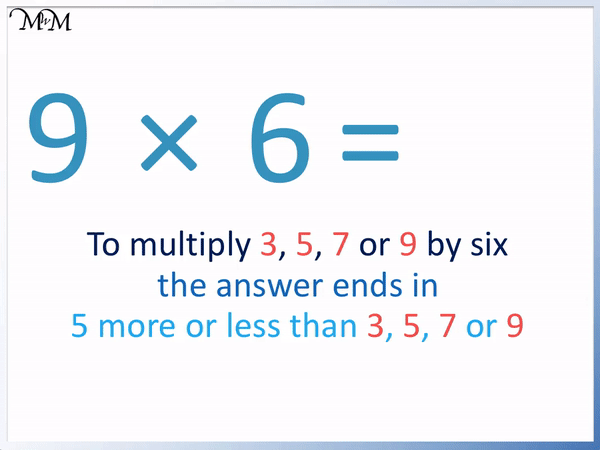We can see that 9 × 6 = 54.

Again, even if we added 5 to 9 we would get 14, which ends in a 4.

We now have a trick for both the even numbers and the odd numbers multiplied by 6.

We just have 10 × 6, 11 × 6 and 12 × 6 to go.

We will start with 10 × 6. Before learning the 6 times table, it is already easiest to know the ten times table.

To multiply a whole number by 10, we simply put a zero on the end.

6 × 10 = 60 and so, 10 × 6 = 60.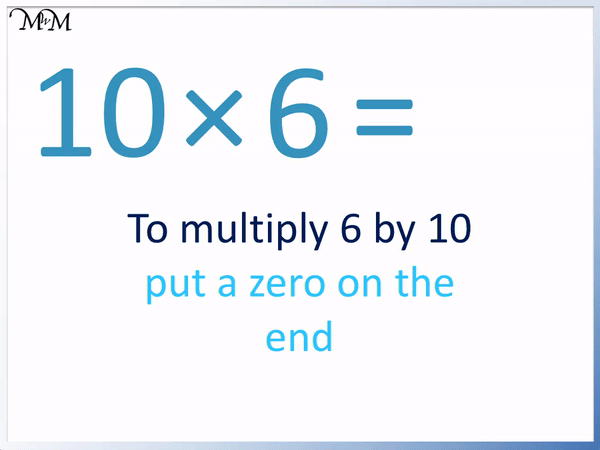Before learning the 6 times table, it is also recommended to learn the 11 times table.

To multiply a single digit by 11, simply repeat the digit.

6 × 11 = 66, we repeat 6.

Therefore 11 × 6 = 66 as well.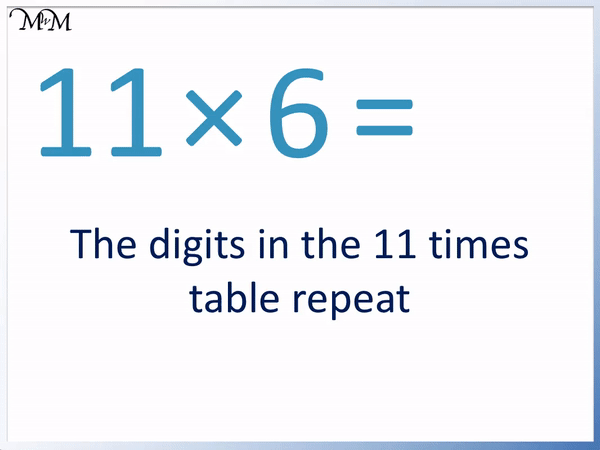Both 10 × 6 = 60 and 11 × 6 = 11 should not be too difficult to remember.

Finally, we have 12 × 6.

The easiest way to multiply by 12 is to multiply by 10, multiply by 2 and then add the results together.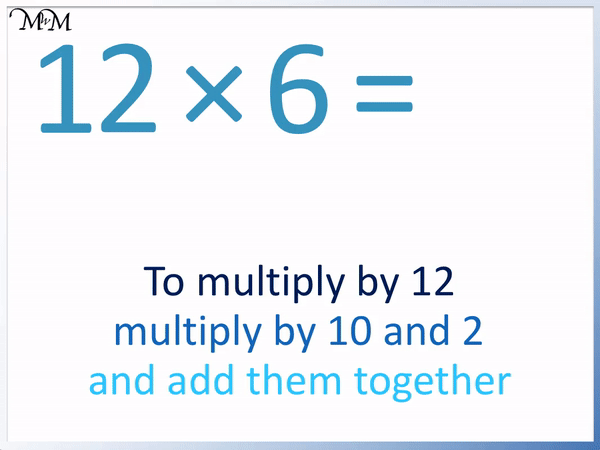6 × 10 = 60

6 × 2 = 12.

60 + 12 = 72 and so, 6 × 12 = 72.

Therefore 12 × 6 = 72 as well.

When in doubt, we can always count up in sixes to make the 6 times table.

If we remember one answer to the 6 times table, we can find the next number along by adding 6 to it.

We can see that the numbers in the 6 times table repeat the pattern of ending in 6, 2, 8, 4 and then 0.This pattern can be used to help us remember what number comes next and it can help us add 6 to the previous numbers.

For example, if we remember 4 × 6 = 24,then we can work out 5 × 6.

24 ends in a 4 and this comes before 0 in the pattern of 6, 2, 8, 4, 0.

We know that 5 × 6 ends in a 0.

We can use 4 × 6 = 24 to work out that 5 × 6 = 30.

If we remember that 7 × 6 = 42, we can use this to work out 8 × 6.

42 ends in a 2, which is before 8 in the pattern 6, 2, 8, 4, 0.

This can help us work out that 8 × 6 = 48.Now try our lesson on Multiplication by Partitioning where we learn how to multiply larger numbers by partitioning them into tens and units.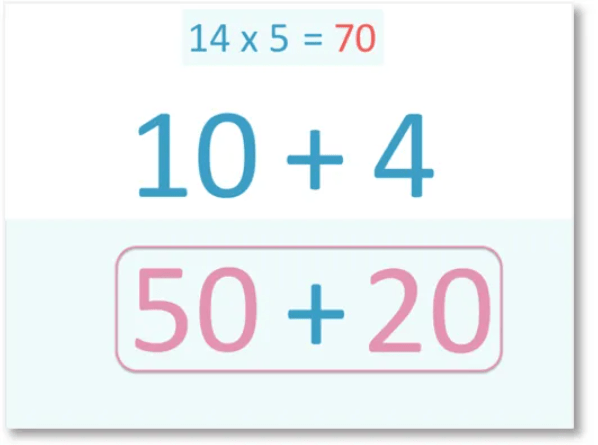error: Content is protected !!